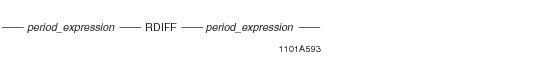# 15.00 - RDIFF - Teradata Database

## Teradata Database SQL Functions, Operators, Expressions, and Predicates

Product
Release Number
15.00
Content Type
Programming Reference
Publication ID
B035-1145-015K
Language
English (United States)

## RDIFF

### Purpose

Returns the portion of the first Period expression that exists from the end of the second Period expression when the Period expressions overlap. When the Period expressions overlap but there is no portion of the first Period expression from the end of the second Period expression or if the Period expressions do not overlap, RDIFF returns NULL. If either Period expression is NULL, RDIFF returns NULL.

### Syntaxwhere:

 Syntax element... Specifies... period_expression any expression that evaluates to a Period data type. Note: The Period expressions specified must be comparable. Implicit casting to a Period data type is not supported.

### Result Value

• Assuming p1 and p2 are comparable Period expressions, p1 RDIFF p2 returns PERIOD(END(p2), END(p1)) if p1 OVERLAPS p2 is TRUE and END(p1) is greater than END(p2). If either Period expression is NULL, p1 OVERLAPS p2 is FALSE, or END(p1) is not greater than END(p2), the result is NULL.
• If the Period expressions have PERIOD(TIME[(n)] [WITH TIME ZONE]) or PERIOD(TIMESTAMP[(n)] [WITH TIME ZONE]) data types but have different precisions, the result has the higher of the two precisions. If one of the Period expressions contains time zones and the other does not, the result contains a time zone for each element. The result time zones are evaluated using the following rules:
• If both Period expressions have a time zone, the time zone displacement of a result bound is obtained from the corresponding bound of the Period expressions as defined by the Period value constructor that follows.
• If only one of the Period expressions has a time zone, the other Period expression is considered to be at the current session time zone and the result is computed as follows.
• Assuming p1 and p2 are Period expressions and the result element type as determined above is rt, the result of p1 RDIFF p2 is as follows if p1 OVERLAPS p2 is TRUE:

`PERIOD(`
`  CASE WHEN CAST(END(p1) AS rt) > CAST(END(p2) AS rt)`
`     THEN CAST(END(p2) AS rt)`
`     ELSE NULL END,`
`  CASE WHEN CAST(END(p1) AS rt) > CAST(END(p2) AS rt)`
`     THEN CAST(END(p1) AS rt)`
`     ELSE NULL END)`

Internally, Period values are saved in UTC and the OVERLAPS operator is evaluated using these UTC represented formats and the RDIFF operation is performed if they overlap.

### Format and Title

The format is the default format for the resulting Period data type.

### Error Conditions

If either expression is not a Period expression, an error is reported.

If the Period expressions are not comparable, an error is reported.

### Example

RDIFF is used to find the right difference of the first Period expression with the second Period expression.

Assume the query is executed on the following employee table where period1 and period2 are PERIOD(DATE) columns:

`   SELECT ename, period2 RDIFF period1 FROM employee;`
`   ename   period1                        period2`
`   -----   ----------------------------   ----------------------------`
`   Adams   ('2005-02-03', '2006-02-03')   ('2005-02-03', '2006-02-03')`
`   Mary    ('2005-04-02', '2006-01-03')   ('2005-02-03', '2006-02-03')`
`   Jones   ('2001-01-02', '2003-03-05')   ('2002-03-05', '2004-10-07')`
`   Randy   ('2006-01-02', '2007-03-05')   ('2004-03-07', '2005-10-07')`
`   Simon   ?                              ('2005-02-03', '2005-07-27')`

The result is:

`   ename   (period2 RDIFF period1)`
`   -----   ----------------------------`
`   Adams   ?`
`   Mary    ('2006-01-03', '2006-02-03')`
`   Jones   ('2003-03-05', '2004-10-07')`
`   Randy   ?`
`   Simon   ?`